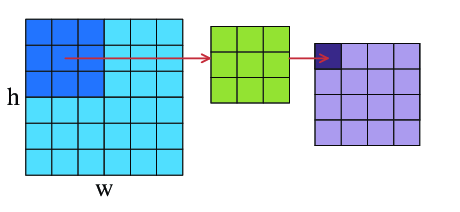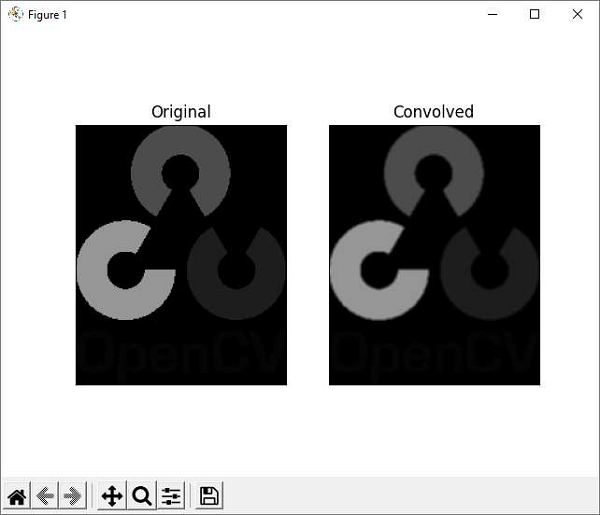# OpenCV Python - Image Filtering

An image is basically a matrix of pixels represented by binary values between 0 to 255 corresponding to gray values. A color image will be a three dimensional matrix with a number of channels corresponding to RGB.

Image filtering is a process of averaging the pixel values so as to alter the shade, brightness, contrast etc. of the original image.

By applying a low pass filter, we can remove any noise in the image. High pass filters help in detecting the edges.

The OpenCV library provides cv2.filter2D() function. It performs convolution of the original image by a kernel of a square matrix of size 3X3 or 5X5 etc.

Convolution slides a kernel matrix across the image matrix horizontally and vertically. For each placement, add all pixels under the kernel, take the average of pixels under the kernel and replace the central pixel with the average value.

Perform this operation for all pixels to obtain the output image pixel matrix. Refer the diagram given below −The cv2.filter2D() function requires input array, kernel matrix and output array parameters.

## Example

Following figure uses this function to obtain an averaged image as a result of 2D convolution. The program for the same is as follows −

import numpy as np
import cv2 as cv
from matplotlib import pyplot as plt
kernel = np.ones((3,3),np.float32)/9
dst = cv.filter2D(img,-1,kernel)
plt.subplot(121),plt.imshow(img),plt.title('Original')
plt.xticks([]), plt.yticks([])
plt.subplot(122),plt.imshow(dst),plt.title('Convolved')
plt.xticks([]), plt.yticks([])
plt.show()


## Output## Types of Filtering Function

Other types of filtering function in OpenCV includes −

• BilateralFilter − Reduces unwanted noise keeping edges intact.

• BoxFilter − This is an average blurring operation.

• GaussianBlur − Eliminates high frequency content such as noise and edges.

• MedianBlur − Instead of average, it takes the median of all pixels under the kernel and replaces the central value.